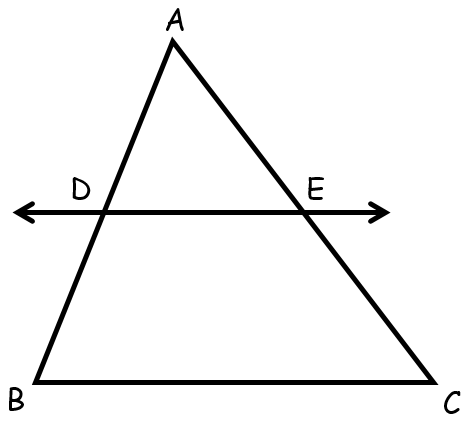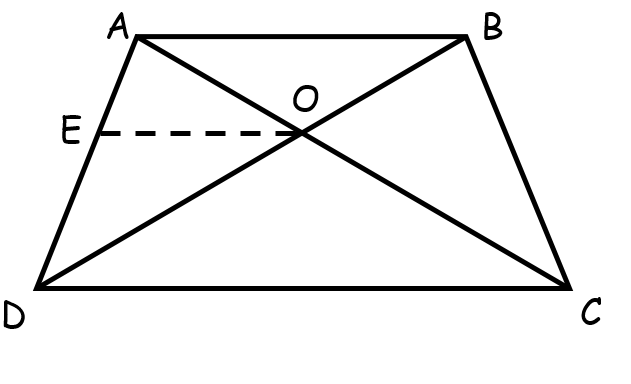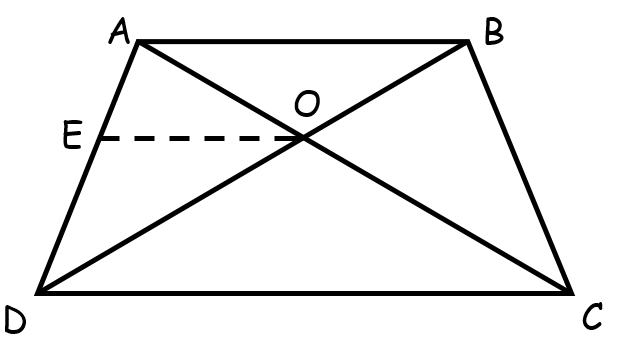# vs.eyeandcontacts.com

## Chapter 6 Triangles Exercise 6.1

Question 1: In Fig. 6.17, (i) and (ii), DE || BC. Find EC in (i) and AD in (ii).For this question we will use Basic Proportionality theorem.

i) According to Basic Proportionality Theorem,
= 1.5/3 = 1/EC
= 1.5(EC) = 1(3)
= 1.5 EC = 3
= EC = 3/1.5
= EC = 2

Therefore, EC = 2 cm

ii) According to Basic Proportionality Theorem,

Question 2: E and F are points on the sides PQ and PR respectively of a ∆PQR. For each of the following cases, state whether EF || QR:
(i) PE = 3.9 cm, EQ = 3 cm, PF = 3.6 cm and FR = 2.4 cm
(ii) PE = 4 cm, QE = 4.5 cm, PF = 8 cm and RF = 9 cm
(iii) PQ = 1.28 cm, PR = 2.56 cm, PE = 0.18 cm and PF = 0.36 cm

For this question we will use converse of Basic Proportionality Theorem,

i)To prove that EF || QR in case (i), we have to show that PE/EQ = PF/FR
= 3.9/3 = 3.6/2

Since 3.9/3 3.6/2.4. Therefore by converse of Basic Proportionality Theorem, EF is not parallel to QR.

ii)To prove that EF || QR in case (ii), we have to show that PE/EQ = PF/FR
= 4/4.5 = 8/9
= 4/4.5 = 4/4.5

Since 4/4.5 = 8/9. Therefore by converse of Basic Proportionality Theorem, EF is parallel to QR.

iii)To prove that EF || QR in case (iii), we have to show that PE/EQ = PF/FR
= PE/PQ = PF/PR    (by corollary of Basic Proportionality Theorem)
= 0.18/1.28 = 0.36/2.56
= 18/128 = 36/256    (multiplying with 100)

Simplifying both sides,
= 9/64 = 18/128
= 9/64 = 9/64

Since 0.18/1.28 = 0.36/2.56. Therefore by converse of Basic Proportionality Theorem, EF is parallel to QR.

Question 3: In Fig. 6.18, if LM || CB and LN || CD, prove that AM/AB = AN/AD.Considering ∆ABC,
Since ML || BC,
∴ By Basic Proportionality Theorem,
AM/AB = AL/AC    …(1)

Since LN || CD
∴ By Basic Proportionality Theorem,

From (1) and (2)

Hence proved.

Question 4: In Fig. 6.19, DE || AC and DF || AE. Prove that BF/FE = BE/EC.Considering ∆ABE with DF || AE.
Since DF || AE
By Basic Proportionality Theorem,
BD/DA = BF/FE    …(1)

Considering ∆ABC with DE || AC.
Since DE || AC
By Basic Proportionality Theorem,
BD/DA = BE/EC    …(2)

From (1) and (2)
BF/FE = BE/EC

Hence proved.

Question 5: In Fig. 6.20, DE || OQ and DF || OR. Show that EF || QR.Considering ΔPQO,
Since ED || QO,
Therefore, by Basic Proportionality Theorem,
PE/QE = PD/DO    ...(1)

Considering ΔPOR,
Since DF || OR,
Therefore, by Basic Proportionality Theorem,
PD/DO = PF/FR    ...(2)

From (1) and (2),
PE/QE = PF/FR

Since, PE/QE = PF/FR, therefore, by converse of Basic Proportionality Theorem, EF || QR.

Hence proved.

Question 6: In Fig. 6.21, A, B and C are points on OP, OQ and OR respectively such that AB || PQ and AC || PR. Show that BC || QR.Considering ΔOPQ,
Since AB || PQ,
Therefore, by Basic Proportionality Theorem,
OA/AP = OB/BQ    ...(1)

Considering ΔOPR,
Since AC || PR,
Therefore, by Basic Proportionality Theorem,
OA/AP = OC/CR    ...(2)

From (1) and (2)
OB/BQ = OC/CR

Since OB/BQ = OC/CR, therefore by converse of Basic Proportionality theorem, BC || QR.

Hence proved

Question 7: Using Theorem 6.1, prove that a line drawn through the mid-point of one side of a triangle parallel to another side bisects the third side. (Recall that you have proved it in Class IX).Given:
DE || BC
D is midpoint of AB

To prove:
E intersects at AC

Since DE || BC,
Therefore, by Basic Proportionality Theorem,
1 = AE/EC    (since D is midpoint of AB)
EC = AE

Since EC = AE, therefore E intersects AC and is the midpoint of AC.

Question 8: Using Theorem 6.2, prove that the line joining the mid-points of any two sides of a triangle is parallel to the third side. (Recall that you have done it in Class IX).Given:
D is midpoint of AB and E is midpoint of AC

To prove:
DE || BC

Since it is given AD/DB = AE/EC
1 = 1    (as D and E are midpoints of AB and AC respectively)

Now,
Since AD/DB = AE/EC, therefore,  by converse of Basic Proportionality Theorem, DE || BC

Hence proved.

Question 9: ABCD is a trapezium in which AB || DC and its diagonals intersect each other at the point O. Show that AO/BO = CO/DO.Given:
Trapezium ABCD where AB || CD and AB & CD intersect each other at O.

To prove:
AO/BO = CO/DO

Construction:
Draw a line EO through O such that EO || DC || AB

Proof:
OE || DC    (by construction)
Therefore, by Basic Proportionality Theorem,
AE/ED = AO/CO    ...(1)

In ΔABD,
OE || AB    (by construction)
Therefore, by Basic Proportionality Theorem,
DE/EA = DO/BO or EA/DE = BO/DO    ...(2)

From (1) and (2)
AO/CO = BO/DO
= (AO)(DO) = (BO)(CO)
= AO/BO = CO/DO

Hence proved.

Question 10: The diagonals of a quadrilateral ABCD intersect each other at the point O such that AO/BO = CO/DO. Show that ABCD is a trapezium.Given:
Quadrilateral ABCD where AC & BD intersect each other at O, such that AO/BO = CO/DO.

To prove:
ABCD is a trapezium

Construction:
Draw a line EO through O such that EO || AB, which meets AD at E.

Proof:
In Δ DAB,
EO || AB    (by construction)
Therefore, by Basic Proportionality Theorem,
DE/EA = DO/OB    ...(1)

Also it is given,
AO/BO = CO/DO
= (AO)(DO) = (CO)(BO)
= AO/CO = BO/DO or CO/AO = DO/BO    ...(2)

From (1) and (2)
DE/EA = CO/AO

Therefore by converse of Basic Proportionality Theorem, EO is parallel to DC also EO is parallel to AB
DC || AB

Since two pair of sides (AB and CD) are parallel to each other, therefore, ABCD is a trapezium.